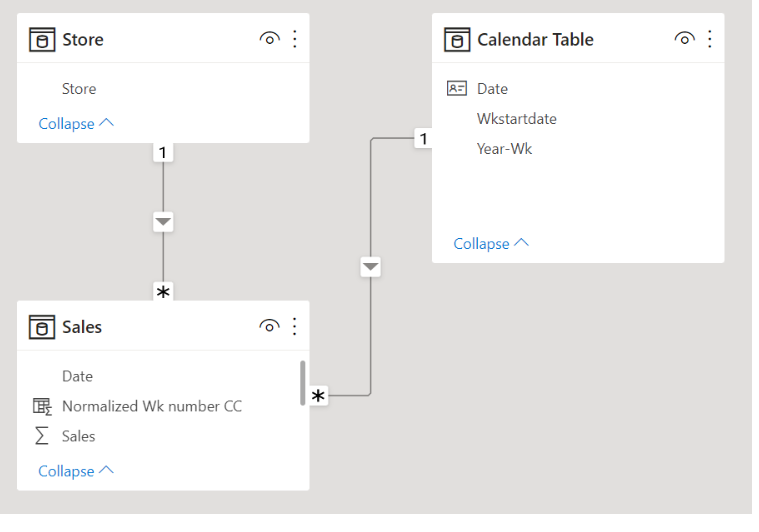cancel
Showing results for
Did you mean:Helper I

## Sales Trend which is normalized

Hi guys,

For example, Staten Island opened in Week 8 of 2021, we would compare sales of that store from Week 9 2021 onwards with Hyannis that opened in Week 17 of 2022.

I will defenitley appreciate can anyone give solution for this.

Thanks,

Srikanth

1 ACCEPTED SOLUTIONSuper User

Hi,

Please check the attached file and the below.

1. create a dim-calendar table

2. create a relationship between the sales table and dim-calendar table

3. create two calculated columns in the sales table

main point is to create a calculated column = Normalized Wk number CC

``````Normalized Wk number CC =
RANKX (
SUMMARIZE (
FILTER ( Sales, Sales[Store] = EARLIER ( Sales[Store] ) ),
Sales[Startdate of Wk CC]
),
Sales[Startdate of Wk CC],
,
ASC
)
``````

4. create a measure that shows sales total and put it into the visualization that uses one of the newly created calculated columns as the X-axisIf this post helps, then please consider accepting it as the solution to help other members find it faster, and give a big thumbs up.

2 REPLIES 2Super User

Hi,

Please check the attached file and the below.

1. create a dim-calendar table

2. create a relationship between the sales table and dim-calendar table

3. create two calculated columns in the sales table

main point is to create a calculated column = Normalized Wk number CC

``````Normalized Wk number CC =
RANKX (
SUMMARIZE (
FILTER ( Sales, Sales[Store] = EARLIER ( Sales[Store] ) ),
Sales[Startdate of Wk CC]
),
Sales[Startdate of Wk CC],
,
ASC
)
``````

4. create a measure that shows sales total and put it into the visualization that uses one of the newly created calculated columns as the X-axisIf this post helps, then please consider accepting it as the solution to help other members find it faster, and give a big thumbs up.Helper I

Thank you.